V.5 No 2 37 On basic formalism of special theory of relativityFirst of all, we have to mark, it were not Einstein and not Minkowski who made the basic mistake that caused the distorted understanding of 4-D interval. This was A. Poincare who in his work “Dynamics of electron” , two years before the famous Minkowski’s report, introduced the complex 4-D space as follows: “Consider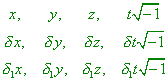(90)
 as coordinates of three points P, P' and P'' in the space of four dimensions. We can easily see, Lorentz transformation is nothing else than the turn in this space about the coordinate origin thought to be stationary. Thus, only six distances between three points P, P' and P'' will differ one from others, or, if we please, two expressions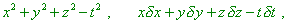(91)
 or four expressions of the same form yielded as the result of any rearrangement of the points P, P'  and P'' ” [24, p. 478]. The mistake was, in complex plane (space), for any complex number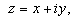(92)
 “the module of a complex number is determined in an unique manner: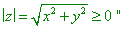(93)
 [25, p. 13]. With it in limits of mathematical tool of the theory of functions of complex variable [25, p. 13],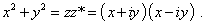(94)
 Thus, if we correctly approach the issue of complex 4-D interval in Minkowski space, its record has to be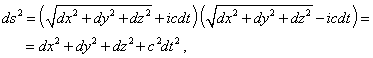(95)
 but in no way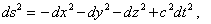(96)
 as we could see it in (91). Hence, (96) under no conditions can determine the interval in the complex plane. And such statements cannot substantiate it: “the form (54) (similar to our (96) - Authors) determines the metric of plane space with the signature like (- - - +), which is called the space of Minkowski who pointed such interpretation convenient” [14, p. 93]. The very record in complex form predetermines the appearance of resulting expressions. But a simple substantiation in the module of complex number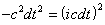(97)
 is incorrect and does not reflect the features of transformations admissible in the complex plane. While relativists in their trivial pursuit of sensations simply copied this definition with accuracy to erroneous claims like this: “The expression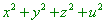(98)
 is invariant with respect to Lorentz transformation and is the quadratic form of coordinates; this suggests an idea to consider it as a squared distance of the world point P (x, y, z, u)  from the coordinate origin, by analogy with related squared distance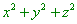(99)
 in usual space. This establishes in 4-D world the geometry (metrics) quite kindred to Euclidean geometry. However, both geometries are not identical, as one of coordinates is imaginary. So, for example, two world points with zero distance not certainly will coincide… Despite this difference in geometrical properties, we can, however, consider Lorentz transformation by an analogy with the coordinate system rotation in R3  as an orthogonal linear transformation of world coordinates and as rotation (imaginary) of the world axes” [6, p. 40]. This is so typical for relativistic approach to physical problems, and Einstein made no secret of it: “Instead to differ real and unreal, we clearly differ the values that belong to the physical system (irrespectively of the choice of coordinate system) and the values depending on the coordinate system” [17, p. 621]. As the result, Pauli, not having time to formulate properly the metric of Minkowski space, had to speak of its shortcomings and of some ‘special’ geometry, which is introduced with identification of concepts of interval and invariant. In fact, the difficulty is connected not with the complex space. In most cases this space can be reduced either to 2-D or to 3-D space, especially in studying inertial motions of a body in frames of SR. There in this complex space acts the self-consistent system of operations with variables and functions. So the mathematical formalism which relativists may use, especially in studying the issues of GR, in such or other way has to be stipulated by operations admissible in complex spaces, especially when in this space they consider conformal maps ([14, chapter 7]). Premising the complex pattern of space and at the same time using ‘as we please’ the transformations being inadmissible from the view of transformation in complex plane, as well as the reference or some feature of signature of this space, with retained definition (i.e., properties, too) of the imaginary unity - can bring only wrong results and discrepancies, and we do see it on the typical example of paradoxical properties of relativistic 4-D interval.

Contents: / 29 / 30 / 31 / 32 / 33 / 34 / 35 / 36 / 37 / 38 / 39 /